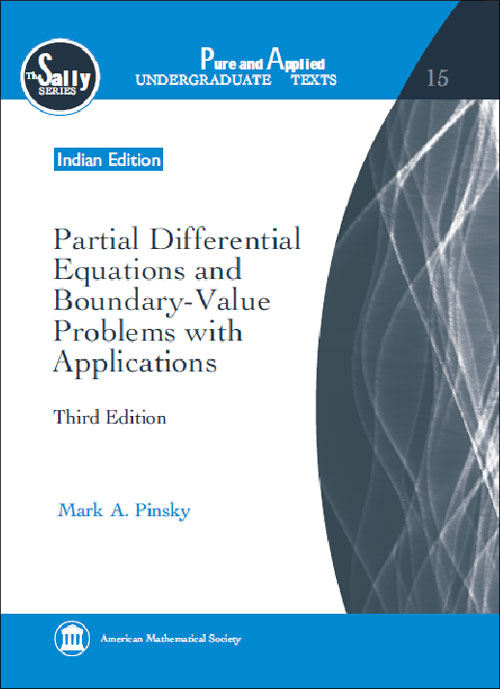Partial Differential Equations and Boundary-Value Problems with Applications
Mark A. Pinsky
Price
1450.00
ISBN
9781470409142
Language
English
Pages
544
Format
Paperback
Dimensions
180 x 240 mm
Year of Publishing
2013
Territorial Rights
Restricted
Imprint
Universities Press

Out Of Stock

Catalogues

Write-up for the back cover, brochure and website Building on the basic techniques of separation of variables and Fourier series, the book presents the solution of boundary-value problems for basic partial differential equations: the heat equation, wave equation, and Laplace equation, considered in various standard coordinate systems--rectangular, cylindrical, and spherical. Each of the equations is derived in the three-dimensional context; the solutions are organized according to the geometry of the coordinate system, which makes the mathematics especially transparent. Bessel and Legendre functions are studied and used whenever appropriate throughout the text. The notions of steady-state solution of closely related stationary solutions are developed for the heat equation; applications to the study of heat flow in the earth are presented. The problem of the vibrating string is studied in detail both in the Fourier transform setting and from the viewpoint of the explicit representation (d''Alembert formula). Additional chapters include the numerical analysis of solutions and the method of Green''s functions for solutions of partial differential equations. The exposition also includes asymptotic methods (Laplace transform and stationary phase).

With more than 200 working examples and 700 exercises (more than 450 with answers), the book is suitable for an undergraduate course in partial differential equations.

Mark A. Pinsky, Northwestern University, Evanston, IL

0 Preliminaries
0.1 Partial Differential Equations
0.2 Separation of Variables
0.3 Orthogonal Functions
1 Fourier Series
1.1 Definitions and Examples
1.2 Convergence of Fourier Series
1.3 Uniform Convergence and the Gibbs Phenomenon
1.4 Parseval's Theorem and Mean Square Error
1.5 Complex Form of a Fourier Series
1.6 Sturm-Liouville Eigenvalue Problems

2 Boundary-Value Problems in Rectangular Coordinates
2.1 The Heat Equation
2.2 Homogeneous Boundary Conditions
2.3 Non-homogeneous Boundary Conditions
2.4 The Vibrating String
2.5 Applications of Multiple Fourier Series

3 Boundary-Value Problems in Cylinderical Coordinates
3.1 Laplace's Equation and Applications
3.2 Bessel Functions
3.4 Heat Flow in the Infinite Cylinder
3.5 Heat Flow in the Finite Cylinder

4 Boundary-Value Problems in Spherical Coordinates
4.1 Spherically Symmetric Solutions
4.2 Legendre Functions and Spherical Bessel Functions
4.3 Laplace's Equation in Spherical Coordinates

5 Fourier Transforms and Applications
5.1 Basic Properties of the Fourier Transform
5.2 Solution of the Heat Equation for an Infinite Rod
5.3 Solutions of the Wave Equation and Laplace's Equation
5.4 Solution of the Telegraph Equation

6 Asymptotic Analysis
6.1 Asymptotic Analysis of the Factorial Function
6.2 Integration by Parts
6.3 Laplace's Method
6.4 The Method of Stationary Phase
6.5 Asymptotic Expansions
6.6 Asymptotic Analysis of the Telegraph Equation

7 Numerical Analysis
7.1 Numerical Analysis of Ordinary Differential Equations
7.2 The One-Dimensional Heat Equation
7.3 Equations in Several Dimensions
7.4 Variational Methods
7.5 Approximate Methods of Ritz and Kantorovich
7.6 Orthogonality Methods

8 Green's Functions
8.1 Green's Functions for Ordinary Differential Equations
8.2 The Three-Dimensional Poisson Equation
8.3 Two-Dimensional Problems
8.4 Green's Function for the Heat Equation
8.5 Green's Function for the Wave Equation

Appendixes
Index